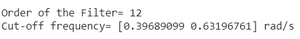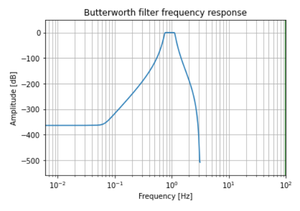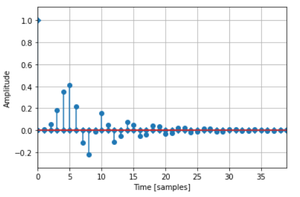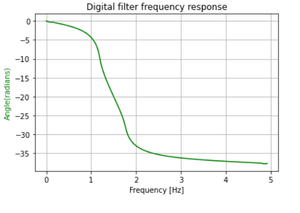# Digital Band Pass Butterworth Filter in Python

• Last Updated : 17 Dec, 2020

In this article, we are going to discuss how to design a Digital Low Pass Butterworth Filter using Python. The Butterworth filter is a type of signal processing filter designed to have a frequency response as flat as possible in the pass band. Let us take the below specifications to design the filter and observe the Magnitude, Phase & Impulse Response of the Digital Butterworth Filter.

## What is Digital Bandpass Filter?

A band-pass filter is a filter that passes frequencies within a range and rejects frequencies outside that range.

Attention geek! Strengthen your foundations with the Python Programming Foundation Course and learn the basics.

To begin with, your interview preparations Enhance your Data Structures concepts with the Python DS Course. And to begin with your Machine Learning Journey, join the Machine Learning - Basic Level Course

## How it’s different from Highpass & Lowpass:

The main difference can be spotted by observing the magnitude response of the Band Pass Filter. The passband of the filter is of a specific range, which means that the only signal’s within this range can be passed by the Bandpass filter. Any signal which doesn’t fall within the specified range is rejected by the filter.

The specifications are as follows:

• Sampling rate of 40 kHz
• Pass band edge frequencies are 1400 Hz & 2100 Hz
• Stop band edge frequencies are 1050 Hz & 2450 Hz
• Pass band ripple of 0.4 dB
• Minimum stop band attenuation of 50 dB

We will plot the magnitude, phase, and impulse response of the filter.

Step-by-step Approach:

Before starting, first, we will create a user-defined function to convert the edge frequencies, we are defining it as convert() method.

## Python3

 `# explicit function to convert``# edge frequencies``def` `convertX(f_sample, f):``    ``w ``=` `[]``     ` `    ``for` `i ``in` `range``(``len``(f)):``        ``b ``=` `2``*``((f[i]``/``2``)``/``(f_sample``/``2``))``        ``w.append(b)`` ` `    ``omega_mine ``=` `[]`` ` `    ``for` `i ``in` `range``(``len``(w)):``        ``c ``=` `(``2``/``Td)``*``np.tan(w[i]``/``2``)``        ``omega_mine.append(c)`` ` `    ``return` `omega_mine`

Now below are the steps:

Step 1: Importing all the necessary libraries.

## Python3

 `# import required modules ``import` `numpy as np ``import` `matplotlib.pyplot as plt ``from` `scipy ``import` `signal ``import` `math`

Step 2: Define variables with the given specifications of the filter.

## Python3

 `# Specifications of Filter`` ` `# sampling frequency``f_sample ``=` `7000`` ` `# pass band frequency``f_pass ``=` `[``1400``, ``2100``]`` ` `# stop band frequency``f_stop ``=` `[``1050``, ``2450``]`` ` `# pass band ripple``fs ``=` `0.5`` ` `# Sampling Time``Td ``=` `1`` ` `# pass band ripple``g_pass ``=` `0.4`` ` `# stop band attenuation``g_stop ``=` `50`

Step 3: Building the filter using signal.buttord() function.

## Python3

 `# Conversion to prewrapped analog ``# frequency ``omega_p``=``convertX(f_sample,f_pass)``omega_s``=``convertX(f_sample,f_stop)``   ` `# Design of Filter using signal.buttord ``# function ``N, Wn ``=` `signal.buttord(omega_p, omega_s, ``                       ``g_pass, g_stop, ``                       ``analog``=``True``) ``   ` `   ` `# Printing the values of order & cut-off frequency``# N is the order ``print``(``"Order of the Filter="``, N) `` ` `# Wn is the cut-off freq of the filter ``print``(``"Cut-off frequency= {:} rad/s "``.``format``(Wn)) ``   ` `   ` `# Conversion in Z-domain ``   ` `# b is the numerator of the filter & a is``# the denominator ``b, a ``=` `signal.butter(N, Wn, ``'bandpass'``, ``True``) ``z, p ``=` `signal.bilinear(b, a, fs) `` ` `# w is the freq in z-domain & h is the ``# magnitude in z-domain ``w, h ``=` `signal.freqz(z, p, ``512``)`Step 4: Plotting the Magnitude Response.

## Python3

 `# Magnitude Response``plt.semilogx(w, ``20``*``np.log10(``abs``(h)))``plt.xscale(``'log'``)``plt.title(``'Butterworth filter frequency response'``)``plt.xlabel(``'Frequency [Hz]'``)``plt.ylabel(``'Amplitude [dB]'``)``plt.margins(``0``, ``0.1``)``plt.grid(which``=``'both'``, axis``=``'both'``)``plt.axvline(``100``, color``=``'green'``)``plt.show()`Step 5: Plotting the Impulse Response.

## Python3

 `# Impulse Response``imp ``=` `signal.unit_impulse(``40``)``c, d ``=` `signal.butter(N, ``0.5``)``response ``=` `signal.lfilter(c, d, imp)`` ` `plt.stem(np.arange(``0``, ``40``), imp, markerfmt``=``'D'``, use_line_collection``=``True``)``plt.stem(np.arange(``0``, ``40``), response, use_line_collection``=``True``)``plt.margins(``0``, ``0.1``)`` ` `plt.xlabel(``'Time [samples]'``)``plt.ylabel(``'Amplitude'``)``plt.grid(``True``)``plt.show()`Step 6: Plotting the Phase Response.

## Python3

 `# Frequency Response``fig, ax1 ``=` `plt.subplots()``ax1.set_title(``'Digital filter frequency response'``)``ax1.set_ylabel(``'Angle(radians)'``, color``=``'g'``)``ax1.set_xlabel(``'Frequency [Hz]'``)`` ` `angles ``=` `np.unwrap(np.angle(h))`` ` `ax1.plot(w``/``2``*``np.pi, angles, ``'g'``)``ax1.grid()``ax1.axis(``'tight'``)``plt.show()`Below is the complete program based on the above approach:

## Python3

 `# User-defined function to convert the ``# values of edge frequencies``def` `convertX(f_sample,f):``  ``w``=``[]``   ` `  ``for` `i ``in` `range``(``len``(f)):``    ``b``=``2``*``((f[i]``/``2``)``/``(f_sample``/``2``))``    ``w.append(b)`` ` `  ``omega_mine``=``[]`` ` `  ``for` `i ``in` `range``(``len``(w)):``    ``c``=``(``2``/``Td)``*``np.tan(w[i]``/``2``)``    ``omega_mine.append(c)`` ` `  ``return` `omega_mine`` ` `# Importing Libraries``import` `numpy as np``import` `matplotlib.pyplot as plt``from` `scipy ``import` `signal``import` `math``  ` `# Specifications of Filter ``   ` `# sampling frequency ``f_sample ``=``7000``   ` `# pass band frequency ``f_pass ``=``[``1400``,``2100``] ``   ` `# stop band frequency ``f_stop ``=``[``1050``,``2450``]  ``   ` `# pass band ripple ``fs ``=` `0.5``   ` `# Sampling Time ``Td ``=` `1`  `   ` ` ``# pass band ripple ``g_pass ``=` `0.4` `   ` `# stop band attenuation ``g_stop ``=` `50`  ` ` `# Conversion to prewrapped analog``# frequency ``omega_p``=``convertX(f_sample,f_pass)``omega_s``=``convertX(f_sample,f_stop)``   ` `# Design of Filter using signal.buttord ``# function ``N, Wn ``=` `signal.buttord(omega_p, omega_s, ``                       ``g_pass, g_stop, ``                       ``analog``=``True``) ``   ` `   ` `# Printing the values of order & cut-off frequency``# N is the order ``print``(``"Order of the Filter="``, N)  ``# Wn is the cut-off freq of the filter ``print``(``"Cut-off frequency= {:} rad/s "``.``format``(Wn)) ``   ` `   ` `# Conversion in Z-domain ``   ` `# b is the numerator of the filter & a is ``# the denominator ``b, a ``=` `signal.butter(N, Wn, ``'bandpass'``, ``True``) ``z, p ``=` `signal.bilinear(b, a, fs) `` ` `# w is the freq in z-domain & h is the magnitude``# in z-domain ``w, h ``=` `signal.freqz(z, p, ``512``)`` ` `# Magnitude Response``plt.semilogx(w, ``20``*``np.log10(``abs``(h)))``plt.xscale(``'log'``)``plt.title(``'Butterworth filter frequency response'``)``plt.xlabel(``'Frequency [Hz]'``)``plt.ylabel(``'Amplitude [dB]'``)``plt.margins(``0``, ``0.1``)``plt.grid(which``=``'both'``, axis``=``'both'``)``plt.axvline(``100``, color``=``'green'``)``plt.show()`` ` `# Impulse Response``imp ``=` `signal.unit_impulse(``40``)``c, d ``=` `signal.butter(N, ``0.5``)``response ``=` `signal.lfilter(c, d, imp)``plt.stem(np.arange(``0``, ``40``),imp,markerfmt``=``'D'``,use_line_collection``=``True``)``plt.stem(np.arange(``0``,``40``), response,use_line_collection``=``True``)``plt.margins(``0``, ``0.1``)``plt.xlabel(``'Time [samples]'``)``plt.ylabel(``'Amplitude'``)``plt.grid(``True``)``plt.show()`` ` `# Frequency Response``fig, ax1 ``=` `plt.subplots()``ax1.set_title(``'Digital filter frequency response'``)``ax1.set_ylabel(``'Angle(radians)'``, color``=``'g'``)``ax1.set_xlabel(``'Frequency [Hz]'``)``angles ``=` `np.unwrap(np.angle(h))``ax1.plot(w``/``2``*``np.pi, angles, ``'g'``)``ax1.grid()``ax1.axis(``'tight'``)``plt.show()`My Personal Notes arrow_drop_up